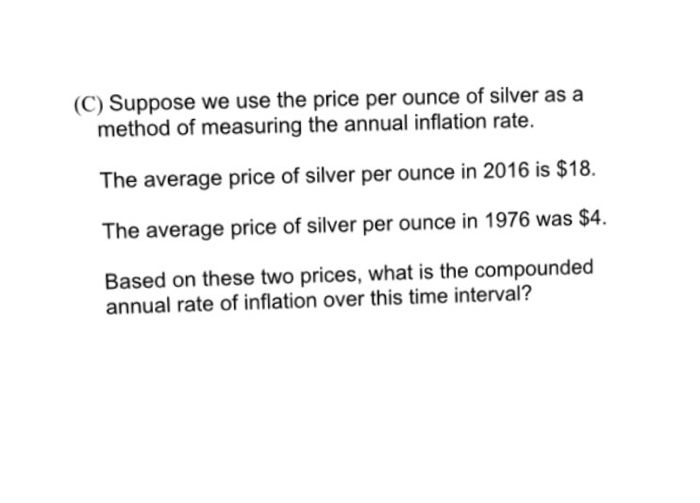### Create an Account

Home / Questions / Suppose we use the price per ounce of silver as a method of measuring the annual inflation...

# Suppose we use the price per ounce of silver as a method of measuring the annual inflation rate. The average price of silver per ounce in 2016 is \$18. The average price of silver per ounce

Suppose we use the price per ounce of silver as a method of measuring the annual inflation rate. The average price of silver per ounce in 2016 is \$18. The average price of silver per ounce in 1976 was \$4 Based on these two prices, what is the compounded annual rate of inflation over this time interval?Jul 04 2021 View more View LessSubscribe To Get Solution